Categories

# Programmer interview challenge 1: Anagram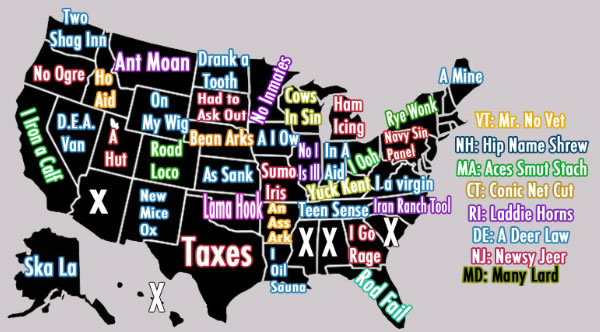An anagram is a word, phrase, or name that can be formed by rearranging the letters in another word, phrase, or name. For example, iceman is an anagram of cinema, and vice versa. Ignoring spaces and capitalizations, “Florida” is an anagram of “rod fail”.

“Anagram” is a common programming challenge that I’ve seen issued to prospective developers in technical interviews: Write a program or function that can tell if two given words are anagrams of each other. Here’s how you solve it.

## The general idea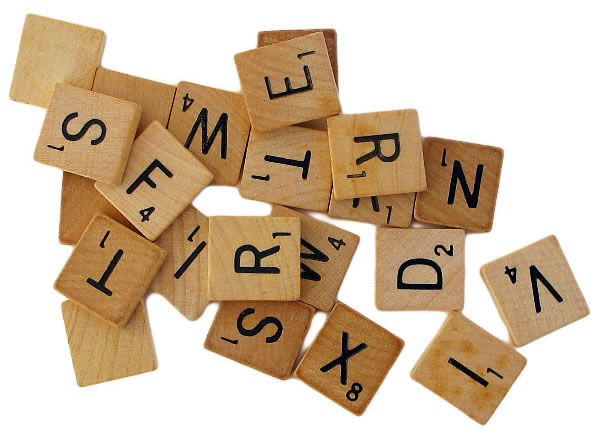One solution to the problem is hinted at in the definition of “anagram”. Let’s look at it again:

An anagram is a word, phrase, or name that can be formed by rearranging the letters in another word, phrase, or name.

The word rearranging should be your hint. Somehow, the solution should involve rearranging the letters of both words so that you can compare them. Another word for rearranging is reordering, and when you encounter that word in programming, you should think of sorting. That’s where the solution lies:

If two words are anagrams of each other, sorting each word’s letters into alphabetical order should create two identical words. For example, if you sort the letters in cinema and iceman into alphabetical order, both will be turned into aceimn.

With that in mind, let’s try writing an anagram-detecting function. Given two strings, it should return `true` if they’re anagrams of each other, and `false` otherwise.

## The Python version

This assumes that you’ve got Python and pytest installed on your system. I recommend installing the Individual Edition of the Anaconda Python distribution, followed by entering `pip install pytest` at the commend line.

Let’s do this the test-first way. We’ll write the test first, and then write the code. This means that we’ll want to create two files:

• anagram.py, which will contain the actual code of the solution, and
• test_anagram.py, which will contain test for that code.

Here’s the code for the test:

```import pytest
from anagram import anagram

def test_simple_anagram():
assert anagram('iceman', 'cinema'), "'cinema' is an anagram of 'iceman'."```

…and here’s the code for the initial iteration of the anagram function:

```def anagram(first_word, second_word):
return False```

To run the test, enter `pytest` at the command line. You should see output that looks like this: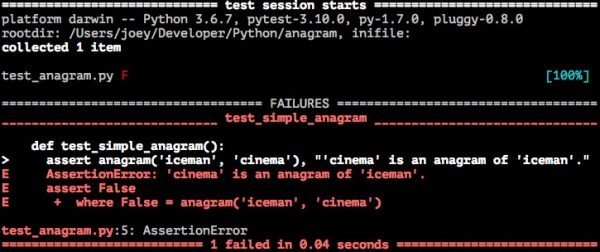Now that we have a failing test, let’s write code to make it pass.

Sorting the letters in a string is Python can be done by using a couple of methods:

• `sorted()`, which when given a string, returns an array containing that string’s letters in ascending order. For example, `sorted('cinema')` returns `['a', 'c', 'e', 'i', 'm', 'n']`.
• `join()`, which when given a string and an array, returns a string where the elements of the array are joined by the given string. For example, `'*'.join(['a', 'b', 'c'])` returns `'a*b*c'`.

Here’s my code:

```def anagram(first_word, second_word):
first_word_sorted = ''.join(sorted(first_word))
second_word_sorted = ''.join(sorted(second_word))
return first_word_sorted == second_word_sorted```

Running `pytest` now gives a passing test: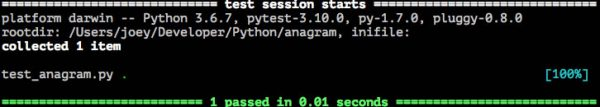Let’s now deal with cases with capitalization and spaces. Ideally, the `anagram()` method should treat “Florida” and “rod fail” as anagrams. We’ll specify this in the test:

```import pytest
from anagram import anagram

def test_simple_anagram():
assert anagram('iceman', 'cinema'), "'cinema' is an anagram of 'iceman'."

def test_complex_anagram():
assert anagram('Florida', 'rod fail'), "'rod fail', if you ignore spaces and capitalization, is an anagram of 'Florida'."```

Running `pytest` yields these results: 1 failed test and 1 passed test…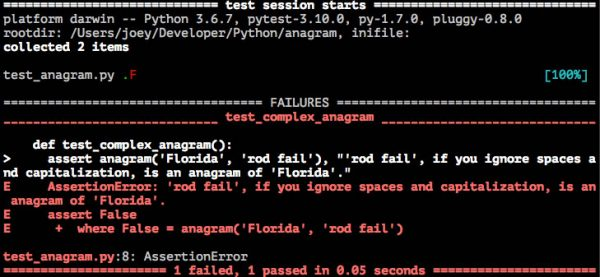We can fix this through the use of another two methods:

• `lower()`, which when applied to a string, converts all its letters to lowercase. For example, `'RADAR'.lower()` returns `'radar'`.
• `lstrip()`, which when applied to a string, removes any whitespace characters from the left side. Since the space character has a lower value than any letter in the alphabet, it will always be the leftmost character in a string whose characters have been sorted into ascending order.

Here’s my revised code:

```def anagram(first_word, second_word):
first_word_lowercase = first_word.lower()
first_word_sorted = ''.join(sorted(first_word_lowercase)).lstrip()
second_word_lowercase = second_word.lower()
second_word_sorted = ''.join(sorted(second_word_lowercase)).lstrip()
return first_word_sorted == second_word_sorted```

Running `pytest` now shows that all the tests pass: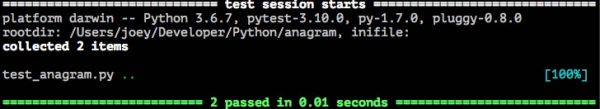Just to be safe, let’s add a test to make sure than `anagram()` returns `False` when given two strings that are not anagrams of each other:

```import pytest
from anagram import anagram

def test_simple_anagram():
assert anagram('iceman', 'cinema'), "'cinema' is an anagram of 'iceman'."

def test_complex_anagram():
assert anagram('Florida', 'rod fail'), "'rod fail', if you ignore spaces and capitalization, is an anagram of 'Florida'."

def test_non_anagram():
assert anagram('ABC', 'xyz') == False, "'ABC' and 'xyz' are not anagrams."```

All test pass when `pytest` is run: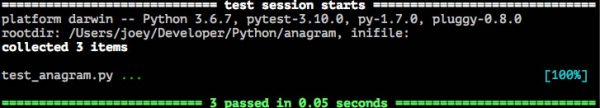And trying all sorts of pairs of strings confirms what the test tells us: `anagram()` works!

```# All of these return True
anagram('Oregon', 'no ogre')
anagram('North Dakota', 'drank a tooth')
anagram('Wisconsin', 'cows in sin')

# All of these return False
anagram('Florida', 'i oil sauna') # Try Louisiana
anagram('New York', 'on my wig') # Try Wyoming
anagram('Georgia', 'navy sin panel') # Try Pennsylvania
```

…and there you have it!

Next: Implementing `anagram()` in JavaScript, Swift, and possibly other languages.

## 8 replies on “Programmer interview challenge 1: Anagram”

[…] In the previous article, I wrote about a challenge that’s often given to programmers at a tech int… Write a program that determines if two words are anagrams. I posted a solution in Python; check it out here. In this article, we’ll refactor the Python code and write a JavaScript implementation. […]

[…] In the first article, I wrote a basic Python solution. In the second article, I refined the Python solution and then based a JavaScript solution on it. In this article, I’ll present a couple of Swift solutions. […]

[…] In the first article, I wrote a basic Python solution. In the second article, I refined the Python solution and then based a JavaScript solution on it. In the third article, I showed a couple of Swift implementations — one simple, one hardcore. In this article, I’ll present Ruby and C# solutions. […]

[…] Programmer interview challenge 1: Anagram […]

[…] Programmer interview challenge 1: Anagram […]

[…] Programmer interview challenge 1: Anagram […]

[…] Programmer interview challenge 1: Anagram […]

[…] Programmer interview challenge 1: Anagram […]